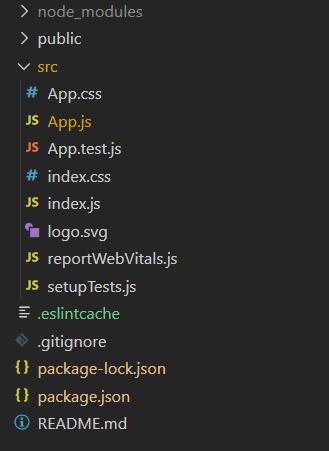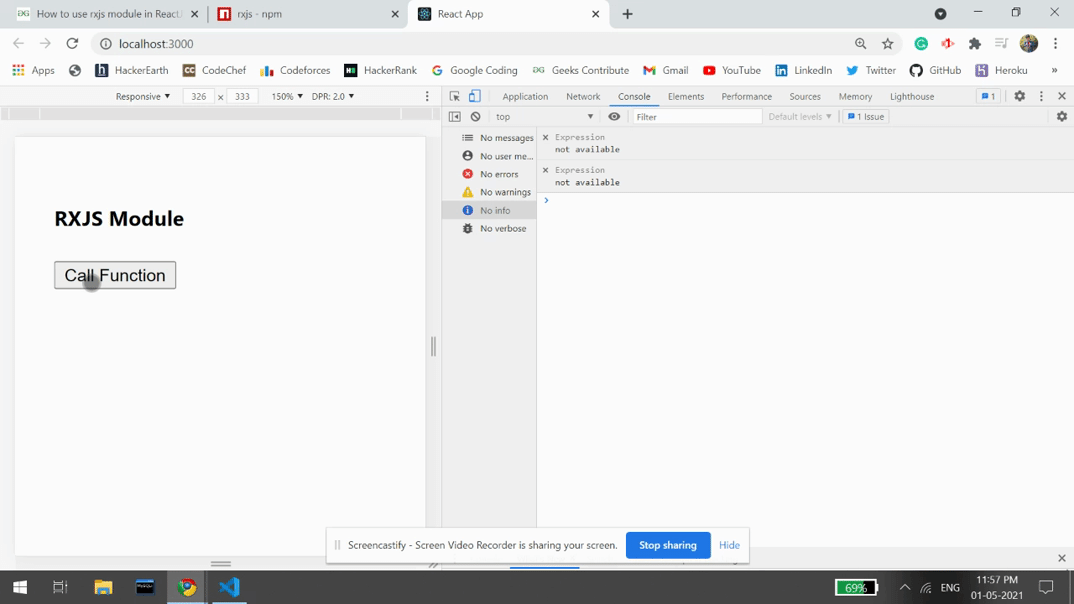# How to use rxjs module in ReactJS ?

• Last Updated : 21 May, 2021

Rxjs stands for Reactive Extensions for JavaScript. This module provides the implementation of observable type to work with reactive programming which is an asynchronous programming paradigm. We can use the following approach in ReactJS to use the rxjs module.

Approach: In the following example, we have used the Rxjs module to demonstrate how we can use it in our ReactJS application. We can create a user-defined printData function which has a range function starting from 1 to 50, and it filters the number whose modulo 2 is equal to 1 and map its to multiply itself, and then we have printed the resulted number in our console screen. We have a button on UI and if the user clicks, this printData function is called.

Creating React Application And Installing Module:

• Step 1: Create a React application using the following command:

`npx create-react-app foldername`
• Step 2: After creating your project folder i.e. folder name, move to it using the following command:

`cd foldername`
• Step 3: After creating the ReactJS application, Install the required module using the following command:

`npm install rxjs`

Project Structure: It will look like the following.Project Structure

Example: Now write down the following code in the App.js file. Here, App is our default component where we have written our code.

## App.js

 `import React from ``'react'``;``import { range } from ``"rxjs"``;``import { map, filter } from ``"rxjs/operators"``;`` ` `const printData = () => {``  ``range(1, 50).pipe(filter(x => x % 2 === 1),``               ``map(x => x * x))``    ``.subscribe((item) => {``      ``console.log(``"Item: "``, item)``    ``});``}`` ` `export ``default` `function` `App() {``  ``return` `(``    ``
``      ``

RXJS Module

``      ``
``        ````      ``
``    ``
``  ``);``}`

Step to Run Application: Run the application using the following command from the root directory of the project:

`npm start`

Output: Now open your browser and go to http://localhost:3000/, you will see the following output:Reference: https://www.npmjs.com/package/rxjs

My Personal Notes arrow_drop_up